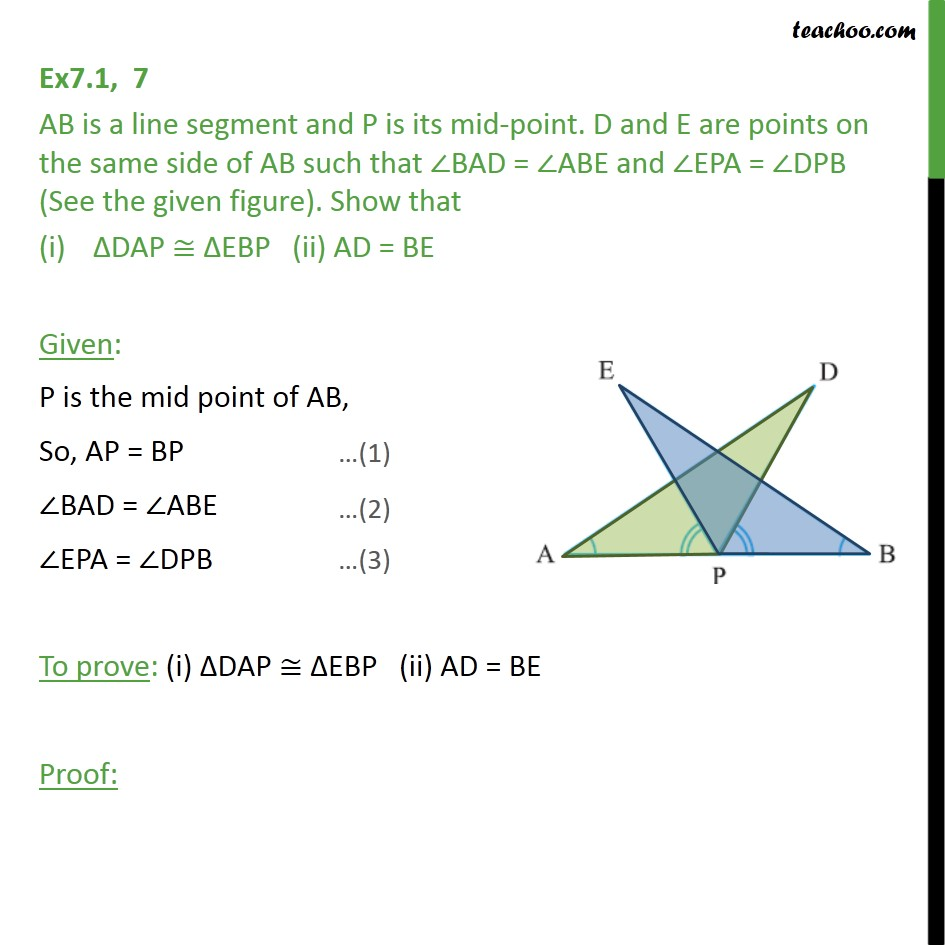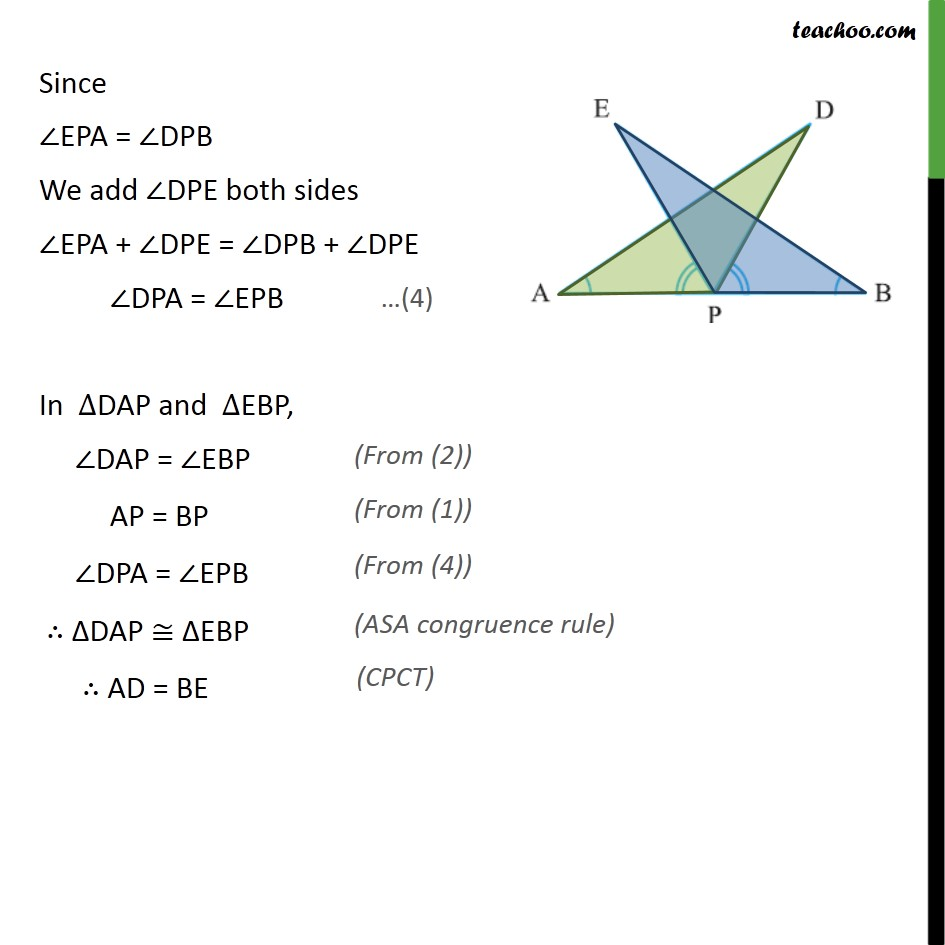Ex 7.1

Chapter 7 Class 9 Triangles
Serial order wiseGet live Maths 1-on-1 Classs - Class 6 to 12

### Transcript

Ex7.1, 7 AB is a line segment and P is its mid-point. D and E are points on the same side of AB such that BAD = ABE and EPA = DPB (See the given figure). Show that DAP EBP (ii) AD = BE Given: P is the mid point of AB, So, AP = BP BAD = ABE EPA = DPB To prove: (i) DAP EBP (ii) AD = BE Proof: Since EPA = DPB We add DPE both sides EPA + DPE = DPB + DPE DPA = EPB In DAP and EBP, DAP = EBP AP = BP DPA = EPB DAP EBP AD = BE